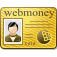# Game theory test

Replenishment date: 26.01.2014
Content: 40126230749783.docx (40.12 KB)
️Automatic issue of goods ✔️
Sales:
12
Refunds:
0
Reviews:
0
Views:
263SellerSeller:
alevtina_sar
Rating:
1,24
Report a violation
Description
Question 1. How is game theory applicable in economics?

1. Game theory in economics is applicable only to the development of organizational structures and incentive systems.
2. Game theory in economics is applicable not only to solving general economic problems, but also to analyze the strategic problems of enterprises, the development of organizational structures and incentive systems.
3. Game theory in economics is applicable only to the analysis of incentive systems.

Question 2. When did game theory appear?

1. The publication in 1904 of the monograph by J. Neumann and O. Morgenstern "Game theory and economic behavior" is considered to be the moment of the birth of game theory.
2. The publication in 1944 of the monograph by J. Neumann and O. Morgenstern "Game theory and economic behavior" is considered to be the moment of the birth of game theory.
3. The publication in 1914 of O. Morgenstern's monograph "Game Theory and Economic Behavior" is considered to be the moment of birth of game theory.

Question 3. What subject areas are key in game theory?

1. Subject areas such as management tasks.
2. Subject areas such as strategic behavior, competition, cooperation, risk and uncertainty are key in game theory and are directly related to management tasks.
3. Subject areas such as strategic behavior, competition.

Question 4. Who and when considered the tasks of production and pricing in an oligopoly?

1. Problems of production and pricing in an oligopoly, which later became textbook examples of game theory, were considered in the XNUMXth century by J. Bertrand.
2. Problems of production and pricing in an oligopoly, which later became textbook examples of game theory, were considered in the XNUMXth century by A. Cournot and J. Bertrand.
3. Problems of production and pricing in conditions of oligopoly, which later became textbook examples of game theory, were considered in the XX century by A. Cournot and J. Bertrand.

Question 5. Who and when put forward the idea of ​​the mathematical theory of conflict of interest?

1. At the beginning of the XII century. E. Lasker, E. Cermelo, E. Borel put forward the idea of ​​a mathematical theory of conflict of interest.
2. At the beginning of the XX century. E. Lasker, E. Cermelo, E. Borel put forward the idea of ​​a mathematical theory of conflict of interest.
3. At the beginning of the XIX century. E. Lasker, E. Cermelo, E. Borel put forward the idea of ​​a mathematical theory of conflict of interest.

Question 6. Who and when were the first to set out the mathematical aspects and applications of game theory?

1. Mathematical game theory has its origins in neoclassical economics. For the first time, the mathematical aspects and applications of the theory were presented in the classic 1904 book by John von Neumann "Theory of Games and Economic Behavior".
2. Mathematical game theory originates from neoclassical economics. For the first time, the mathematical aspects and applications of the theory were presented in the classic 1944 book by John von Neumann and Oskar Morgenstern "Theory of Games and Economic Behavior".
3. Mathematical game theory originates from neoclassical economics. For the first time, the mathematical aspects and applications of the theory were presented in the classic 1914 book by John von Neumann and Oskar Morgenstern "Theory of Games and Economic Behavior".

Question 7. What is the contribution of J. Nash to game theory?

1. In his writings, J. Nash developed the principles of "production dynamics". Nash develops analytical methods in which all participants benefit
2. In his writings, J. Nash developed the principles of "management dynamics". Nash develops methods of analysis in which all participants either win or fail. These situations are called "Nash equilibrium", or "non-cooperative equilibrium", in a situation the parties use the optimal strategy, which leads to the creation of a stable equilibrium. It is beneficial for the players to maintain this balance, since any change will worsen their situation. These works by J. Nash made
Recommended